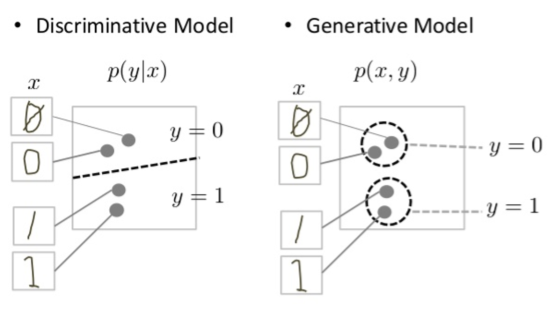Source: Deep Learning on MediumGenerative model is probably one of the interesting and growing fields of machine learning due to its capabilities. One such well-known algorithm is Generative Adversarial Network. Let us delve into this algorithm and try to implement this into lines of code using Python and Tensorflow. (Sufficient background in machine learning, calculus, and experience in Tensorflow are needed)

## What is Generative Model?

Generative model is a type of models that focuses more at finding out how data is generated in terms of a probabilistic model. So that by sampling from this model, we can generate new “synthesis data” that indistinguishable from the real data. But it has to be probabilistic, meaning that the model has to include a stochastic element that influences samples generated.

Generative model is the counterpart of more-popular discriminative model. The latter learns a function that maps an input to an output using a labeled dataset, i.e: the model learns to draw a “boundary” between classes. Examples of discriminative model are k-nearest neighbor, random forest, support vector machine, and linear regression. Figure 1 below illustrates the difference between generative and discriminative model.

From the picture above, we can conclude that discriminative model’s goal is to calculate the possibility of data x belongs to class y, while generative model outputs the probability of data x and class y at the same time.

At least there are 3 reasons why researches in generative model are active:

• Computer scientist, in general, should not be content with only being able to classifying data, but also a complete understanding of how the data was generated
• It is likely that generative modelling will be central to driving future developments of fields in machine learning, e.g: in reinforcement learning, an agent could learn from its own imaginary environment rather than computer simulation or real world
• By owning the ability to generate such dataset, it is one step closer to be comparable to human’s intelligence

## What is GAN?

Generative Adversarial Network(GAN) is a deep-learning-based generative model that introduced by Ian Goodfellow in 2014. As the name suggests, the model contains two opposing models as an approach to generate new dataset.

The two adversarial models are multi layer perceptrons, called generator and discrimantor. Generator is used to generate new plausible datasets, while discriminator tries to classify dataset as real (observation) or fake (generated).

During the training phase, both models are simultaneously trained together with different objectives. Discriminator is merely a classification networks which goals to maximize the probability of assigning the correct label, generated or real. On the other hand, generator takes input of noise variable and represent a mapping to data space so that the generated samples can “fool” discriminator. Such “adversarial training” is where this model get its name from.

Mathematical-wise, GAN objective functions is divided into two: with D(x) as discriminator output for real image, D(G(z)) as output for generated images, 1-D(G(z)) as probability of misclassified generated ones, and log(x) as their respective loss function, generator model goal is to minimize its error by applying gradient descent, while discriminator’s is to maximize its predictive power, hence it is ascending its stochastic gradient. The ideal outcome of this phase is when generator’s samples are indistinguishable from real data and discriminator outputs 1/2, thus it can be discarded.

## What is DCGAN

DCGAN, short for Deep Convolutional GAN is an improvement of existing generative model formalized in 2015. The motivation behind this algorithm is GAN is known for its unstability to train, leading to nonsensical outputs generators resulted.

There are 4 main approaches used in DCGAN:

1. All convolutional net with strided convolutions generated model, allowing it to learn spatial upsampling, and discriminator
2. Eliminating fully connected layers on top of convolutional features directly connecting the highest convolutional features to the input of generator and output of discriminator proven to work well.
3. Batch normalization application, though its usage is avoided in generator output layer and discriminator input layer
4. reLU activation is used throughout layers in the generator, except output layer which uses tanh function. Whilst in the discriminator, leaky rectified activation worked well.

## DCGAN Implementation

DCGAN can be implemented using Tensorflow and python. Dataset used are MNIST Fashion, can be fetched from here: https://github.com/zalandoresearch/fashion-mnist

Function below yields a generator model that takes dense as input and upsample it several times by using Conv2DTranspose. The model uses batch normalization, strided convolutions, and reLU activation, except in the outermost layer.

Next, discriminator model is generated by calling this function, which is an image classification network with leakyreLU activation function.

Next, loss functions should be defined to evaluate the ability of models during the training stage. Discriminator model is measured by total loss of both real and fake class, generator model on the other hand uses cross entropy for the generated image.

Now that models and their respective loss functions has been declared, it is time to put the pieces altogether. Eager execution is applied, so that each tensors gradient can be traced back and computed before passing these into optimizers. In this example, Adam is used.

The above snippets outputs generated images for each class in fashion MNIST dataset as shown below. Notice that there is a stark contrast between images generated after the first and final iteration where scattered, meaningless points remodeled into cloth-shaped images. Thus, the model is able to generate images much alike to the real ones.